WORKING STRESS

# 1 WORKING STRESS

## 1.1 Problem definition

Determinate the total elongation for a bar of a given cross section.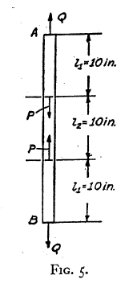Figure 2: Model by Timoshenko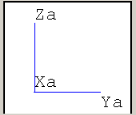Figure 1: Rohr2 model

## 1.2 References (Timoshenko)

S. Timoshenko, Strength of Material, Part I, Elementary Theory and Problems, 3rd Edition, D. Van Nostrand Co., Inc., New York, NY, 1956, Chapter 1.4, pg. 10.

A steel bar, which is classified in three parts, is stressed by four axial forces. At the upper and lower portion, the force-vectors leave the object. The both average force- vectors point to the beam center. Timoshenko gives the following parameters:

• Cross section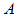• Length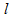• Action force (Q, P)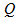,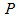• Modulus of Elasticity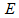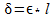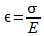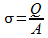,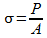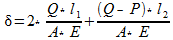Where:

 Variable Description Unit Used Value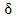Elongation inch 0,008333Length inch 10,00Modulus of Elasticity lbs/ inchÂ² 30000000Cross section inchÂ² 1,00Force lbf 10000Force lbf 5000

Table 1: Overview of the used variables

## 1.3 Model description (ROHR2)

The ROHR2 model consists of three equal vertical parts of a cylindrical carbon steel beam, which are 10 inch long. In this example the bars have the cross section of 1 inchÂ² â‰™6,4516 cmÂ². It is implemented by setting the dimensions with diameter and wall thickness (28,7 mm x 13,6 mm). At the middle of the system it is fixed by a boundary condition (anchor). Following force-directions are defined for several points in the ROHR2 model:

• node A upwards with 10000 lbf

• node 1 downwards with 5000 lbf

• node 3 upwards with 5000 lbf

• node B downwards with 10000 lbf

For this calculation, all loads are scaled, just to recognize the elongation at the ROHR2-output. The applied factor is 1000. In this case the gravity is also disable, so the beam line mass is 0 lb/ ft.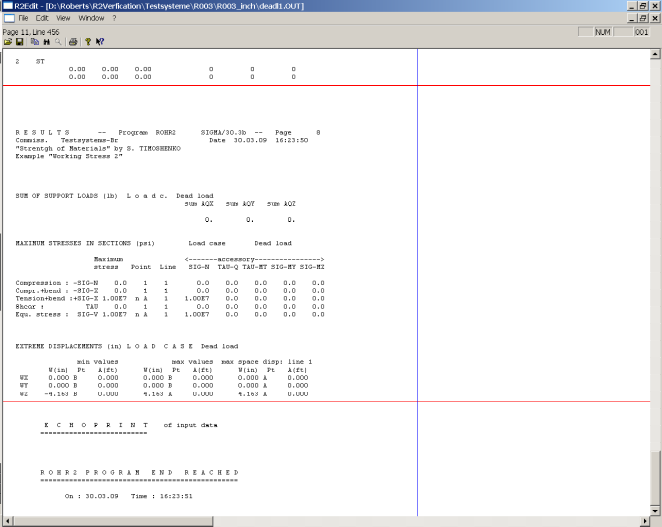Figure 3: Î´ from the Lc Dead Weight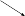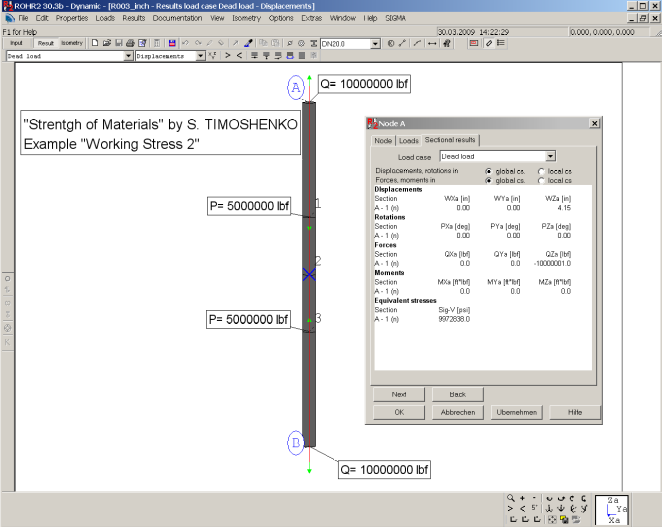Figure 4: Rohr2 model with increased loads and sectional results

## 1.4 Result comparisons

 Value Length [inch] Reference (Timoshenko) [inch] Rohr2 [inch] Difference [%]10 0.008333 0,008326 <0,09

Table 2: Comparison of the total elongation for point A and B

 Value Cross section (Timoshenko) [inchÂ²] Cross section (Rohr 2) [inchÂ²] Difference [%]1,0 0,9999 <0,01

Table 3: Comparison of between required and used cross section

## 1.5 Conclusion

The results are close to the references by Timoshenko. The very small difference appear, only because of the cross section definition, which is not exactly defined enough. In ROHR2 you get at every end point (A, B) just the half displacement. So the user had to multiply the results of the program by a factor 2.

## 1.6 Files

R003_inch.r2w

R003_mm.r2w

R2_stresses_3.ods

SIGMA Ingenieurgesellschaft mbH www.rohr2.com